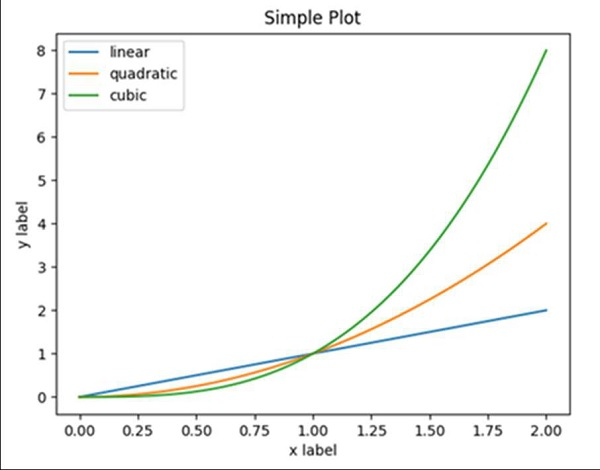# Which is the recommended way to plot â€“ matplotlib or pylab?

pylab is a module that imports matplotlib.pyplot (for plotting) and numpy (for mathematics and working with arrays) in a single namespace.

Although many examples use pylab, it is no longer recommended. For non-interactive plotting, it is suggested to use pyplot to create the figures and then the OO interface for plotting.

## Example

import matplotlib.pyplot as plt
import numpy as np

x = np.linspace(0, 2, 100)

plt.plot(x, x, label='linear')
plt.plot(x, x**3, label='cubic')

plt.xlabel('x label')
plt.ylabel('y label')

plt.title("Simple Plot")

plt.legend()
plt.show()

## Output